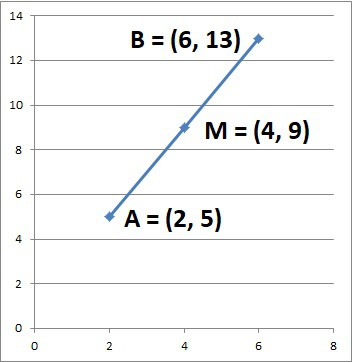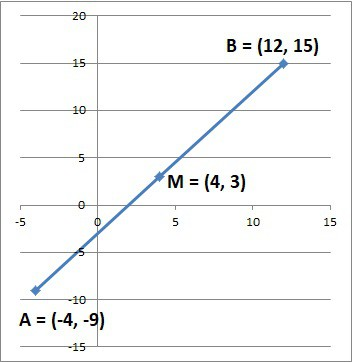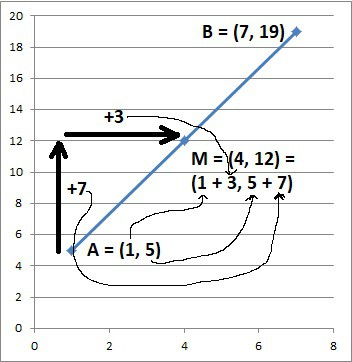# Questions About Midpoints (5 Key Things To Know)

Midpoints are used often in geometry – for example, the midpoint of any diameter of a circle is the center of the circle.  However, there is a lot more to know about midpoints and how to find them.

So, what do you need to know about midpoints?  A midpoint is the middle point of a line segment. A midpoint is the same distance from each endpoint. A midpoint bisects a line segment into two halves, each with the same length (half of the original line segment length). We can use the midpoint formula for line segments in two or three dimensions.

Of course, a midpoint can have negative, decimal, or fractional coordinates.  A ray cannot have a midpoint, since it does not have finite length.

In this article, we’ll talk about midpoints in 2 and 3 dimensions, along some examples of how to find them.  We’ll also answer some common questions about midpoints along the way.

Let’s get started.

## What Is A Midpoint?

A midpoint is the middle point on a line segment.  The midpoint is the point is the same distance from each endpoint of the line segment.

That is, if the endpoints of a line segment are A and B, then the midpoint M is the same distance from A and from B.The mid point of a line segment is half way between the two endpoints (here, M is the same distance from A and B).

### Does A Midpoint Bisect A Line Segment?

A midpoint bisects a line segment into two halves of equal length.  Using the example from before, the line segment from A to M is the same length as the line segment from M to B.

The length of each of these smaller line segments is exactly half of the length of the line segment from A to B.

The center C of a circle is a midpoint for any diameter of the circle.  This makes sense, because the distance from the center of a circle to any point on the circle is the radius (which has a length of half the diameter).

The center R of a rectangle is a midpoint for the two diagonals of the rectangle.

### How To Find The Midpoint Of A Line Segment

To find the midpoint of a line segment, think of taking the “average” of the coordinates in each dimension.  We can write out a formal equation to find the midpoint in two or three dimensions (the midpoint formula).

The Midpoint Formula In Two Dimensions: to find the midpoint of a line segment in two dimensions: if the two endpoints are P1 = (x1, y1) and P2 = (x2, y2), the midpoint M = (xM, yM)has the coordinates given by:

• M = (xM, yM) = ((x1 + x2) / 2, (y1 + y2) / 2)

The Midpoint Formula In Three Dimensions: to find the midpoint of a line segment in three dimensions: if the two endpoints are P1 = (x1, y1, z1) and P2 = (x2, y2, z2), the midpoint M = (xM, yM, zM)has the coordinates given by:

• M = (xM, yM, zM) = ((x1 + x2) / 2, (y1 + y2) / 2, (z1 + z2) / 2)

#### Example 1: Midpoint Of A Line Segment In Two Dimensions (2D Midpoint)

Let’s say we want to find the midpoint of the line segment with endpoints (2, 5) and (6, 13).  Using the midpoint formula with x1 = 2, y1 = 5, x2 = 6, and y2 = 13, we get:

• M = (xM, yM)
• =((x1 + x2) / 2, (y1 + y2) / 2)
• =((2 + 6) / 2, (5 + 13) / 2)
• =((8) / 2, (18) / 2)
• =(4, 9)

So the midpoint (4, 9) is the same distance from (2, 5) and (6, 13).  (You can always verify this with the distance formula).

#### Example 2: Midpoint Of A Line Segment In Three Dimensions (3D Midpoint)

Let’s say we want to find the midpoint of the line segment with endpoints (1, 4, 18) and (7, -6, 12).  Using the midpoint formula with x1 = 1, y1 = 4, z1 = 18, x2 = 7, y2 = -6, and z2 = 12, we get:

• M = (xM, yM)
• =(xM, yM, zM)
• =((x1 + x2) / 2, (y1 + y2) / 2, (z1 + z2) / 2)
• =((1 + 7) / 2, (4 + -6) / 2, (18 + 12) / 2)
• =((8) / 2, (-2) / 2, (30) / 2)
• =(4, -1, 15)

So the midpoint (4, -1, 15) is the same distance from (1, 4, 18) and (7, -6, 12).  (You can always verify this with the distance formula).

### When To Use The Midpoint Formula

We can use the midpoint formula in two cases:

• 1. We know both endpoints of a line segment, and we want to find the midpoint of the line segment.
• 2. We know the midpoint and one endpoint of a line segment, and we want to find the other endpoint of the line segment.

We have seen an example of the first type already.  Let’s take a look at an example of the second type.

#### Example: Use The Midpoint & An Endpoint To Find The Other Endpoint

Let’s say we want to find the other endpoint of the line segment with midpoint (4, 3) and endpoint (12, 15).  Using the midpoint formula with x1 = 12, y1 = 15, xM = 4, yM = 3, we get:

• M = (xM, yM) = ((x1 + x2) / 2, (y1 + y2) / 2)
• (4, 3) = ((12 + x2) / 2, (15 + y2) / 2)

This gives us two equations: one for each coordinate.

For the x-coordinate, we get:

• 4 = (12 + x2) / 2
• 8 = 12 + x2  [multiply both sides by 2]
• -4 = x2

Similarly, for the y-coordinate, we get:

• 3 = (15 + y2) / 2
• 6 = 15 + y2  [multiply both sides by 2]
• -9 = y2

So, the other endpoint has coordinates (x2, y2) = (-4, -9).We can use the midpoint and one endpoint of a line segment to find the other endpoint.

## Can A Midpoint Be A Decimal Or Fraction?

A midpoint can have a decimal or fraction for any of its coordinates: x or y (or z in the case of 3 dimensions).

Let’s look at some examples.

### Example 1: A 2D Midpoint With Fraction Or Decimal Coordinates

Let’s say we want to find the midpoint of the line segment with endpoints (3, 7) and (6, 2).  Using the midpoint formula with x1 = 3, y1 = 7, x2 = 6, and y2 = 2, we get:

• M = (xM, yM)
• =((x1 + x2) / 2, (y1 + y2) / 2)
• =((3 + 6) / 2, (7 + 2) / 2)
• =((9) / 2, (9) / 2)
• =(4.5, 4.5)

So the midpoint (4.5, 4.5) has decimal (or fraction) coordinates for both the x and y coordinates.

### Example 2: A 3D Midpoint With Fraction Or Decimal Coordinates

Let’s say we want to find the midpoint of the line segment with endpoints (2.4, 6.7, 1.8) and (5.9, 1.1, 9.0).  Using the midpoint formula with x1 = 2.4, y1 = 6.7, z1 = 1.8, x2 = 5.9, y2 = 1.1, and z2 = 9.0, we get:

• M = (xM, yM)
• =(xM, yM, zM)
• =((x1 + x2) / 2, (y1 + y2) / 2, (z1 + z2) / 2)
• =((2.4 + 5.9) / 2, (6.7 + 1.1) / 2, (1.8 + 9.0) / 2)
• =((8.3) / 2, (7.8) / 2, (10.8) / 2)
• =(4.15, 3.9, 5.4)

So the midpoint (4.15, 3.9, 5.4)has decimal (or fraction) coordinates for the x, y, and z coordinates.

## Can A Midpoint Be Negative?

A midpoint can have a negative value for any of its coordinates: x or y (or z in the case of 3 dimensions).

Let’s look at an example.

### Example 1: A 2D Midpoint With Negative Coordinates

Let’s say we want to find the midpoint of the line segment with endpoints (-6, -10) and (4, 2).  Using the midpoint formula with x1 = -6, y1 = -10, x2 = 4, and y2 = 2, we get:

• M = (xM, yM)
• =((x1 + x2) / 2, (y1 + y2) / 2)
• =((-6 + 4) / 2, (-10 + 2) / 2)
• =((-2) / 2, (-8) / 2)
• =(-1, -4)

So the midpoint (-1, -4) has negative coordinates for both the x and y coordinates.

## Is Midpoint The Same As Slope?

Midpoint is not the same as slope, since:

• The midpoint of a line segment is the point halfway between the two endpoints.
• The slope of a line segment is the rise over run (vertical change divided by horizontal change, or change in y divided by change in x, between the two points).

However, we can use the rise and run concept to find a midpoint.

### Example: Midpoint From Two Endpoints Using Rise & Run

Consider the two points (x1, y1) = (1, 5) and (x2, y2) = (7, 19).  We can calculate the vertical and horizontal change between these two points:

• Rise = difference in y coordinates = y2 – y1 = 19 – 5 = 14
• Run = difference in x coordinates = x2 – x1 = 7 – 1 = 6

If we add half the rise (14 / 2 = 7) to the y-coordinate of (1, 5) and half the run (6 / 2 = 3) to the x-coordinate of (1, 5), we get:

• x-coordinate of midpoint = 1 + 3 = 4
• y-coordinate of midpoint = 5 + 7 = 12

So, the midpoint is (4, 12).  This is the same result we would get if we used the midpoint formula.We can use one endpoint, along with half the rise and half the run between the two endpoints, to find the midpoint.

Note: when using this method, we must make sure to add half the rise to the first y coordinate (y1) and half the run to the x-coordinate (x1).

Otherwise, you will go in the wrong direction and get a point that is not on the line segment!

Also, be careful not to mix up x and y.  The rise corresponds to y (vertical), and the run corresponds to x (horizontal).

## Does A Ray Have A Midpoint?

A ray does not have a midpoint.  This is because a midpoint implies half of a distance (the length of a line segment), and a ray is not a line segment (and thus does not have a finite length).

A ray has one endpoint and no midpoint.

## Conclusion

Now you know a little more about the midpoint of a line segment, along with how to find it (in 2D or 3D).